Test: Self Inductance - 1

# Test: Self Inductance - 1

Test Description

## 10 Questions MCQ Test Electromagnetic Fields Theory (EMFT) | Test: Self Inductance - 1

Test: Self Inductance - 1 for Electrical Engineering (EE) 2023 is part of Electromagnetic Fields Theory (EMFT) preparation. The Test: Self Inductance - 1 questions and answers have been prepared according to the Electrical Engineering (EE) exam syllabus.The Test: Self Inductance - 1 MCQs are made for Electrical Engineering (EE) 2023 Exam. Find important definitions, questions, notes, meanings, examples, exercises, MCQs and online tests for Test: Self Inductance - 1 below.
Solutions of Test: Self Inductance - 1 questions in English are available as part of our Electromagnetic Fields Theory (EMFT) for Electrical Engineering (EE) & Test: Self Inductance - 1 solutions in Hindi for Electromagnetic Fields Theory (EMFT) course. Download more important topics, notes, lectures and mock test series for Electrical Engineering (EE) Exam by signing up for free. Attempt Test: Self Inductance - 1 | 10 questions in 30 minutes | Mock test for Electrical Engineering (EE) preparation | Free important questions MCQ to study Electromagnetic Fields Theory (EMFT) for Electrical Engineering (EE) Exam | Download free PDF with solutions
 1 Crore+ students have signed up on EduRev. Have you?
Test: Self Inductance - 1 - Question 1

### When the current changes from +2A to -2A in 0.05 second, an emf of 8V is induced in the coil. The coefficient of self inductance of the coil is

Detailed Solution for Test: Self Inductance - 1 - Question 1

Concept:

• Whenever the electric current passing through a coil changes, the magnetic flux linked with it will also change.
• As a result of this, in accordance with Faraday’s laws of electromagnetic induction, an emf is induced in the coil which opposes the change that causes it.
• This phenomenon is called ‘self-induction’ and the emf induced is called back

Induced e.m.f can be given as: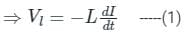Where VL = induced voltage in volts, L = self-inductance of the coil, dl/dt = rate of change of current in ampere/second.

Calculation:
Given:
I1 = +2 Amp, I2 = -2 Amp, dt = 0.05 sec, VL = 8 volt
⇒ dI = I2 - I1 = (-2 - 2) = -4 Amp
From equation 1,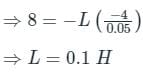Test: Self Inductance - 1 - Question 2

### An emf of 5 V is produced by self - inductance, when the current changes at a steady rate from 3 A to 2 A in 1 ms. The value of self - inductance is

Detailed Solution for Test: Self Inductance - 1 - Question 2

Concept:

• The self-inductance of a coil is defined as the ratio of the magnetic flux and the current flowing through it.
• In a conducting coil, magnetic flux is the product of the magnetic field due to the current flowing in the coil and the area of the coil.
• According to Faraday's law, in a conducting wire, the change in electric current changes the magnetic flux associated with it, and the change in the magnetic flux induces an electric current.
• According to Lenz law, the direction of the induced current is such that the initial change in the electric current is opposed.
• As soon as the change in electric current stops, the induced current also stops flowing.
• The formula to calculate the electromotive force due to induction is,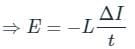Where E is the electromotive force or the potential, t is the time elapsed, L is the self-inductance of the coil and ΔI is the change in the electric current of the coil.

• The S.I unit of self-inductance is Henry(H).

Calculation:
Given: E = 5 V, t = 1 ms = 0.001 s, ΔI = (2A - 3A) = - 1A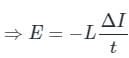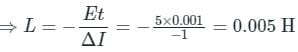Test: Self Inductance - 1 - Question 3

### If we apply law of conversion of energy to electromagnetic induction, electrical energy induced in a conductor comes from

Detailed Solution for Test: Self Inductance - 1 - Question 3

Concept:

• Potential energy: Potential energy is the energy stored within an object, due to the object's position, arrangement or state.
• Heat energy: Heat is the transfer of energy from one system to another, and it can affect the temperature of a singular system.
• Kinetic energy: Kinetic energy is the energy of mass in motion. The kinetic energy of an object is the energy it has because of its motion.
• Radiation energy: Radiation is the emission or transmission of energy in the form of waves or particles through space or through a material medium.

Explanation:

• The work done by moving coil (Change in kinetic energy) induces the electrical energy in the conductor.

This principle is used in electric generators.
So option 3 is correct.

Test: Self Inductance - 1 - Question 4

When number of turns per unit length of a solenoid is doubled, its self inductance becomes:

Detailed Solution for Test: Self Inductance - 1 - Question 4

Concept:

• Self-Induction: Whenever the electric current passing through a coil changes, the magnetic flux linked with it will also change.
• As a result of this, in accordance with Faraday’s laws of electromagnetic induction, an emf is induced in the coil which opposes the change that causes it.
• This phenomenon is called ‘self-induction’ and the emf induced is called back emf, current so produced in the coil is called induced current.

Self-inductance of a solenoid is given by: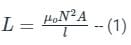Where μo = Absolute permeability, N = Number of turns, l = length of the solenoid, and A = Area of the solenoid.

Explanation:

μo ,I, A are constants. So, we can say that
L = k N2   -- (2)
k is constant, N is the number of turns
L is directly proportional to the square of a number of turns.
If number of turns becomes N' = N2, then inductance
L' = k N'2
⇒ L' = k (2N)2 = k 4 N2 = 4 K N2
⇒ L' = 4 L

So, the inductance is increased by 4 times.
Hence the correct option is 4 times.

Test: Self Inductance - 1 - Question 5

When the length of the solenoid is doubled without any change in the number of turns and the area of the coil.Then its self-inductance will

Detailed Solution for Test: Self Inductance - 1 - Question 5

Concept:

• Whenever the electric current passing through a coil changes, the magnetic flux linked with it will also change.
• As a result of this, in accordance with Faraday’s laws of electromagnetic induction, an emf is induced in the coil which opposes the change that causes it.
• This phenomenon is called ‘self-induction’ and the emf induced is called back emf, current so produced in the coil is called induced current.
• Self-inductance of a solenoid is given by –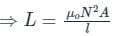Where μo = Absolute permeability, N = Number of turns, l = length of the solenoid, and A = Area of the solenoid.

Explanation

Given - l2 = 2l1

• Self-inductance of a solenoid is given by: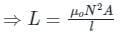• According to the question, the length of the solenoid is doubled without any change in the number of turns and the area of the coil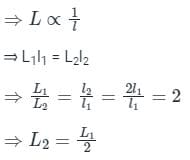Test: Self Inductance - 1 - Question 6

The magnetic potential energy stored in a certain inductor is 25 mJ, when the current in the inductor is 60 mA. This inductor is of inductance:

Detailed Solution for Test: Self Inductance - 1 - Question 6

Concept:

• The coil which stores magnetic energy in a magnetic field is called an inductor.
• The property of an inductor which causes the emf to generate by a change in electric current is called as inductance of the inductor.
• The SI unit of inductance is Henry (H).
• The magnetic potential energy stored in an inductor is given by,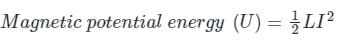Where L is inductance of the inductor and I is current flowing.

Explanation:
Given that:
Magnetic potential energy (U) = 25 mJ = 25 x 10-3 J
Current (I) = 60 mA = 60 x 10-3A
Use the formula: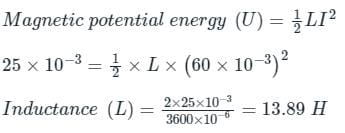Test: Self Inductance - 1 - Question 7

If we increase the current in an inductor, self inductance of the inductor will __________.

Detailed Solution for Test: Self Inductance - 1 - Question 7

Concept:

• Whenever the electric current passing through a coil changes, the magnetic flux linked with it will also change.
• As a result of this, in accordance with Faraday’s laws of electromagnetic induction, an emf is induced in the coil which opposes the change that causes it.
• This phenomenon is called ‘self-induction’ and the emf induced is called back emf, current so produced in the coil is called induced current.
• Self-inductance of a solenoid is given by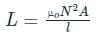Explanation:

• Self-inductance of a solenoid is given by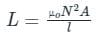• From the above equation, it is clear that the self-inductance of a solenoid depends on the geometry and magnetic permeability of the core material.
• It is independent of current, so if we increase the current in an inductor, there will be no change in the self-inductance of the inductor. Therefore the correct answer is option 3.
Test: Self Inductance - 1 - Question 8

Mutual inductance of two coils can be increased by

Detailed Solution for Test: Self Inductance - 1 - Question 8

Concept:

Mutual Induction:

• Whenever the current passing through a coil or circuit changes, the magnetic flux linked with a neighboring coil or circuit will also change.
• Hence an emf will be induced in the neighboring coil or circuit. This phenomenon is called ‘mutual induction’.
• Mutual induction between the two coils of area A, number of turns N1 and N2 with the length of secondary or primary l is given by –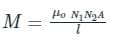Explanation:

• Mutual induction between the two coils of area A, number of turns N1 and N2 with the length of secondary or primary l is given by –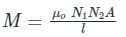Dependence of mutual inductance –

• The number of turns (N1, N2) of both coils.
• Area of the cross-section of coils.
• The magnetic permeability of medium between the coils (μo) or nature of the material on which two coils are wound.
• Length of the coil (l).
• From the above formula, mutual inductance of two coils can be increased by increasing the number of turns in the coils.
Test: Self Inductance - 1 - Question 9

The coefficient of self-inductance of a solenoid is 0.18 mH. If a core of soft iron of relative permeability 900 is inserted, then the coefficient of self-inductance will become nearly

Detailed Solution for Test: Self Inductance - 1 - Question 9

Concept:

Self-Induction:

• Whenever the electric current passing through a coil changes, the magnetic flux linked with it will also change.
• As a result of this, in accordance with Faraday’s laws of electromagnetic induction, an emf is induced in the coil which opposes the change that causes it.
• This phenomenon is called ‘self-induction’ and the emf induced is called back emf, current so produced in the coil is called induced current.
• The self-inductance of a solenoid is given by: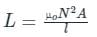Where N = number of turns, A = area of cross-section, l = length of the solenoid and μ0 = absolute permeability

Calculation:
Give - L1 = 0.18 mH = 0.18 x 10-3 H , μ1 = μμ, μ2 = μ and relative permeability (μ) = 900

The self-inductance of a solenoid is given by: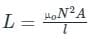Here N, A and l is constant
∴ L ∝ μ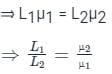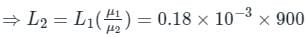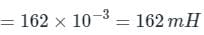Test: Self Inductance - 1 - Question 10

If the magnitude of the applied potential is decreased in the coil, then the direction of the induced current and the current due to applied potential will be

Detailed Solution for Test: Self Inductance - 1 - Question 10

Concept:

Self-induction:

• When current flows through a coil or circuit, the magnetic field is produced and hence a magnetic flux gets associated with this coil or circuit.
• This magnetic flux is directly proportional to the current flowing in the circuit.
• If the current through the coil is changed, the magnetic field also changes, and hence the magnetic flux associated with it also changes, and as a result of this, an emf is induced in the coil or circuit.
• This phenomenon is called self-induction.

Lenz's Law:

• According to this law, the emf will be induced in a coil due to a changing magnetic flux in such a way that it will oppose the cause which has produced it.
• This law states that the induced emf in a conductor due to a changing magnetic flux is such that the magnetic field created by the induced emf opposes the change in a magnetic field.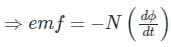where N = number of loops and dϕ =  Change in magnetic flux

The above equation is given by Faraday's law, but the negative sign is a result of Lenz's law.

Explanation:

• If the magnitude of the applied potential is decreased in the coil, the current in the coil also decreases, and due to the self-induction, an emf will get induced in the coil due to which a current will also induce in the coil.
• According to Lenz's law, the direction of induced emf is such that it always opposes change due to which it is produced.
• So here the current in the coil is decreasing, so the induced emf will try to increase it, therefore the direction of the induced current will be the same as the ​current due to applied potential.
• Hence, option 2 is correct.

## Electromagnetic Fields Theory (EMFT)

11 videos|46 docs|62 tests
 Use Code STAYHOME200 and get INR 200 additional OFF Use Coupon Code
Information about Test: Self Inductance - 1 Page
In this test you can find the Exam questions for Test: Self Inductance - 1 solved & explained in the simplest way possible. Besides giving Questions and answers for Test: Self Inductance - 1, EduRev gives you an ample number of Online tests for practice

## Electromagnetic Fields Theory (EMFT)

11 videos|46 docs|62 tests